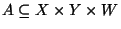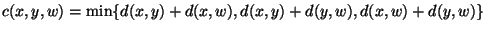Next: MAXIMUM CAPACITY REPRESENTATIVES Up: Weighted Set Problems Previous: MAXIMUM CONSTRAINED PARTITION   Index

### MINIMUM 3-DIMENSIONAL ASSIGNMENT

• INSTANCE: Three sets X, Y, and W and a cost function.
• SOLUTION: An assignment A, i.e., a subsetsuch that every element ofbelongs to exactly one triple in A.
• MEASURE: The cost of the assignment, i.e.,.

• Bad News: Not in APX .
• Comment: The negative result holds even if c is either defined as c(x,y,w) = d(x,y)+d(x,w)+d(y,w) or defined aswhere d is any distance function. In these cases, however, the problem is approximable within 4/3 if d satisfies the triangle inequality. Similar results hold for the k-dimensional problem .
• Garey and Johnson: Weighted version of SP2

Viggo Kann
2000-03-20